# Gravity GRAVITY DEFINED Gravity is the tendency of

• Slides: 10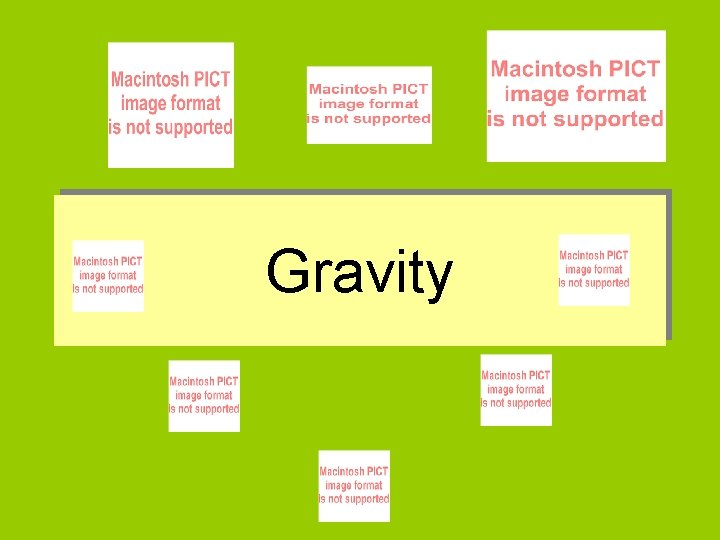GravityGRAVITY DEFINED • Gravity is the tendency of objects with mass to accelerate towards each other • Gravity is one of the four fundamental forces (interactions) in nature.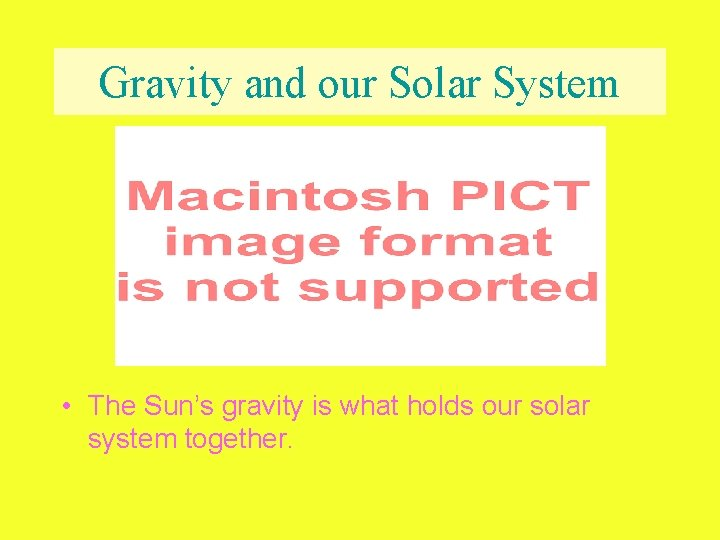Gravity and our Solar System • The Sun’s gravity is what holds our solar system together.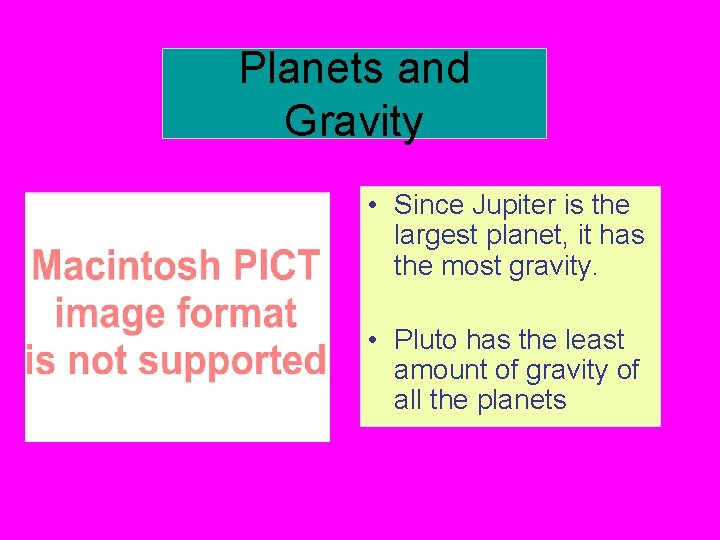Planets and Gravity • Since Jupiter is the largest planet, it has the most gravity. • Pluto has the least amount of gravity of all the planets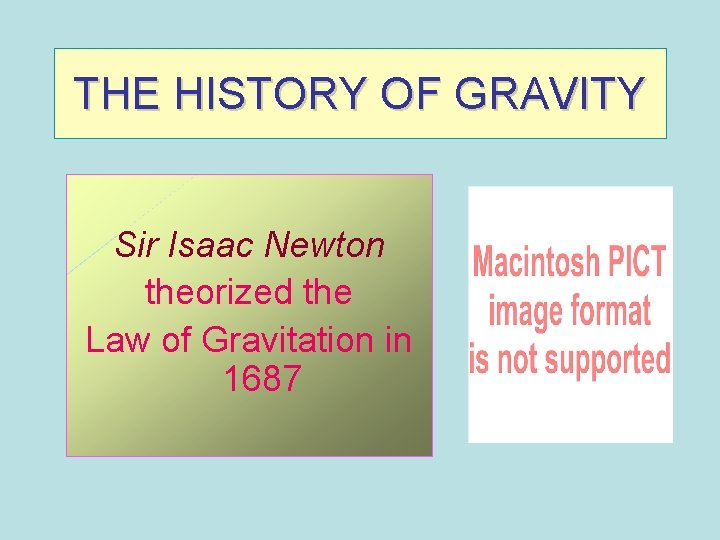THE HISTORY OF GRAVITY Sir Isaac Newton theorized the Law of Gravitation in 1687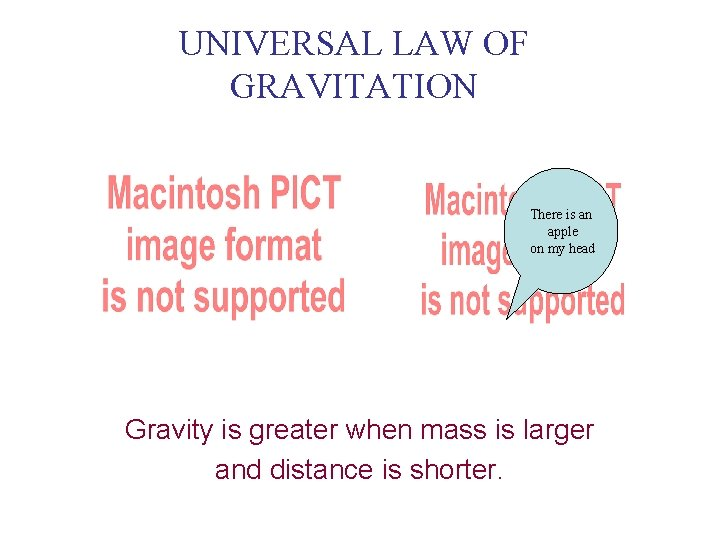UNIVERSAL LAW OF GRAVITATION There is an apple on my head Gravity is greater when mass is larger and distance is shorter.MICROGRAVITY • The further an object is away from another object, the force of gravity is weaker. • The further an object moves from the surface of the Earth, the less gravity it feels from the Earth. • Scientists on the Space Shuttle experience microgravityMass and Weight are two different Properties of Matter MASS v Mass is a physical property of matter that explains how much matter is in an object v Mass does not change when gravity changes WEIGHT v Weight is a force which is calculated by multiplying the acceleration of gravity times mass. v Weight can change when gravity changesMASS • IF YOU PULLED A MOUSE AND AN ELEPHANT WITH THE SAME AMOUNT OF FORCE, THE ELEPHANT WOULD RESPOND LESS TO PULLING EVEN IF HE DIDN’T PULL BACK AT ALL • THAT IS BECAUSE AN ELEPHANT HAS MORE MASS THAN A MOUSE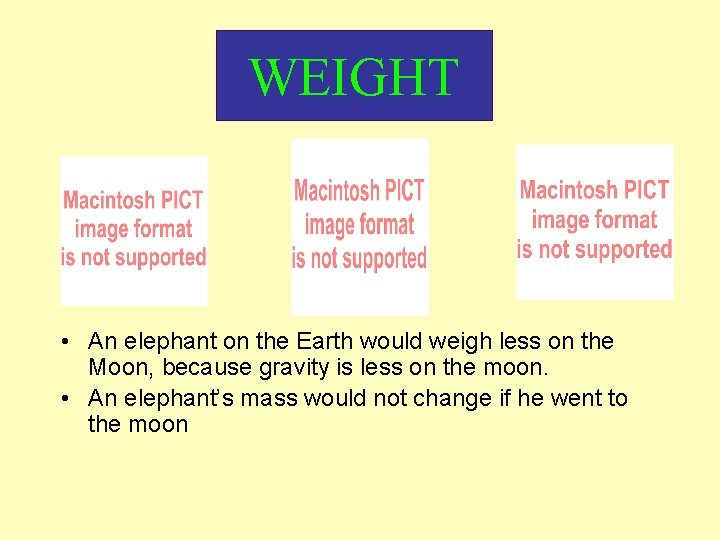WEIGHT • An elephant on the Earth would weigh less on the Moon, because gravity is less on the moon. • An elephant’s mass would not change if he went to the moon